## December 11, 2017

### From the Icosahedron to E8

#### Posted by John BaezHere’s a draft of a little thing I’m writing for the Newsletter of the London Mathematical Society. The regular icosahedron is connected to many ‘exceptional objects’ in mathematics, and here I describe two ways of using it to construct $\mathrm{E}_8$. One uses a subring of the quaternions called the ‘icosians’, while the other uses Du Val’s work on the resolution of Kleinian singularities. I leave it as a challenge to find the connection between these two constructions!

(Dedicated readers of this blog may recall that I was struggling with the second construction in July. David Speyer helped me a lot, but I got distracted by other work and the discussion fizzled. Now I’ve made more progress… but I’ve realized that the details would never fit in the Newsletter, so I’m afraid anyone interested will have to wait a bit longer.)

But blogs are more fun.

### From the Icosahedron to E8

In mathematics, every sufficiently beautiful object is connected to all others. Many exciting adventures, of various levels of difficulty, can be had by following these connections. Take, for example, the icosahedron — that is, the regular icosahedron, one of the five Platonic solids. Starting from this it is just a hop, skip and a jump to the $\mathrm{E}_8$ lattice, a wonderful pattern of points in 8 dimensions! As we explore this connection we shall see that it also ties together many other remarkable entities: the golden ratio, the quaternions, the quintic equation, a highly symmetrical 4-dimensional shape called the 600-cell, and a manifold called the Poincaré homology 3-sphere.

Indeed, the main problem with these adventures is knowing where to stop. The story we shall tell is just a snippet of a longer one involving the McKay correspondence and quiver representations. It would be easy to bring in the octonions, exceptional Lie groups, and more. But it can be enjoyed without these digressions, so let us introduce the protagonists without further ado.

The icosahedron has a long history. According to a comment in Euclid’s Elements it was discovered by Plato’s friend Theaetetus, a geometer who lived from roughly 415 to 369 BC. Since Theaetetus is believed to have classified the Platonic solids, he may have found the icosahedron as part of this project. If so, it is one of the earliest mathematical objects discovered as part of a classification theorem. In any event, it was known to Plato: in his Timaeus, he argued that water comes in atoms of this shape.

The icosahedron has 20 triangular faces, 30 edges, and 12 vertices. We can take the vertices to be the four points

$(0 , \pm 1 , \pm \Phi)$

and all those obtained from these by cyclic permutations of the coordinates, where

$\displaystyle{ \Phi = \frac{\sqrt{5} + 1}{2} }$

is the golden ratio. Thus, we can group the vertices into three orthogonal golden rectangles: rectangles whose proportions are $\Phi$ to 1.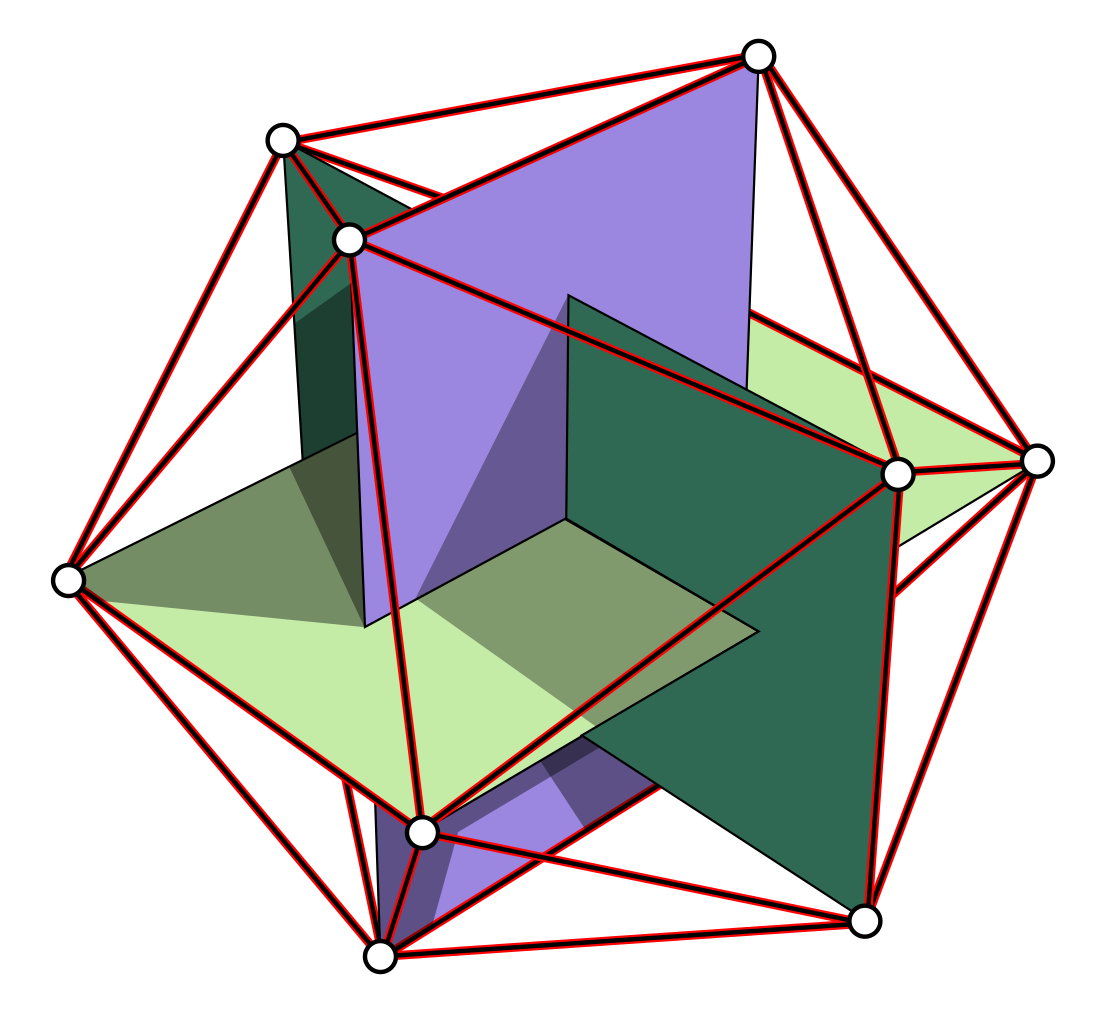In fact, there are five ways to do this. The rotational symmetries of the icosahedron permute these five ways, and any nontrivial rotation gives a nontrivial permutation. The rotational symmetry group of the icosahedron is thus a subgroup of $\mathrm{S}_5$. Moreover, this subgroup has 60 elements. After all, any rotation is determined by what it does to a chosen face of the icosahedron: it can map this face to any of the 20 faces, and it can do so in 3 ways. The rotational symmetry group of the icosahedron is therefore a 60-element subgroup of $\mathrm{S}_5$. Group theory therefore tells us that it must be the alternating group $\mathrm{A}_5$.

The $\mathrm{E}_8$ lattice is harder to visualize than the icosahedron, but still easy to characterize. Take a bunch of equal-sized spheres in 8 dimensions. Get as many of these spheres to touch a single sphere as you possibly can. Then, get as many to touch those spheres as you possibly can, and so on. Unlike in 3 dimensions, where there is “wiggle room”, you have no choice about how to proceed, except for an overall rotation and translation. The balls will inevitably be centered at points of the $\mathrm{E}_8$ lattice!

We can also characterize the $\mathrm{E}_8$ lattice as the one giving the densest packing of spheres among all lattices in 8 dimensions. This packing was long suspected to be optimal even among those that do not arise from lattices — but this fact was proved only in 2016, by the young mathematician Maryna Viazovska [V].

We can also describe the $\mathrm{E}_8$ lattice more explicitly. In suitable coordinates, it consists of vectors for which:

• the components are either all integers or all integers plus $\textstyle{\frac{1}{2}}$, and

• the components sum to an even number.

This lattice consists of all integral linear combinations of the 8 rows of this matrix:

$\left( \begin{array}{rrrrrrrr} 1&-1&0&0&0&0&0&0 \\ 0&1&-1&0&0&0&0&0 \\ 0&0&1&-1&0&0&0&0 \\ 0&0&0&1&-1&0&0&0 \\ 0&0&0&0&1&-1&0&0 \\ 0&0&0&0&0&1&-1&0 \\ 0&0&0&0&0&1&1&0 \\ -\frac{1}{2}&-\frac{1}{2}&-\frac{1}{2}&-\frac{1}{2}&-\frac{1}{2}&-\frac{1}{2}&-\frac{1}{2}&-\frac{1}{2} \end{array} \right)$

The inner product of any row vector with itself is 2, while the inner product of distinct row vectors is either 0 or -1. Thus, any two of these vectors lie at an angle of either 90° or 120° from each other. If we draw a dot for each vector, and connect two dots by an edge when the angle between their vectors is 120° we get this pattern: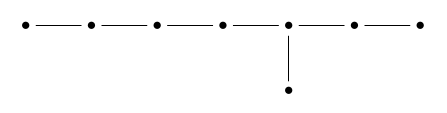This is called the $\mathrm{E}_8$ Dynkin diagram. In the first part of our story we shall find the $\mathrm{E}_8$ lattice hiding in the icosahedron; in the second part, we shall find this diagram. The two parts of this story must be related — but the relation remains mysterious, at least to me.

### The Icosians

The quickest route from the icosahedron to $\mathrm{E}_8$ goes through the fourth dimension. The symmetries of the icosahedron can be described using certain quaternions; the integer linear combinations of these form a subring of the quaternions called the ‘icosians’, but the icosians can be reinterpreted as a lattice in 8 dimensions, and this is the $\mathrm{E}_8$ lattice [CS]. Let us see how this works. The quaternions, discovered by Hamilton, are a 4-dimensional algebra

$\displaystyle{ \mathbb{H} = \{a + b i + c j + d k \colon \; a,b,c,d\in \mathbb{R}\} }$

with multiplication given as follows:

$\displaystyle{i^2 = j^2 = k^2 = -1, }$ $\displaystyle{i j = k = - j i \; and \; cyclic \; permutations }$

It is a normed division algebra, meaning that the norm

$\displaystyle{ |a + b i + c j + d k| = \sqrt{a^2 + b^2 + c^2 + d^2} }$

obeys

$|q q'| = |q| |q'|$

for all $q,q' \in \mathbb{H}$. The unit sphere in $\mathbb{H}$ is thus a group, often called $\mathrm{SU}(2)$ because its elements can be identified with $2 \times 2$ unitary matrices with determinant 1. This group acts as rotations of 3-dimensional Euclidean space, since we can see any point in $\mathbb{R}^3$ as a purely imaginary quaternion $x = b i + c j + d k$, and the quaternion $qxq^{-1}$ is then purely imaginary for any $q \in \mathrm{SO}(3)$. Indeed, this action gives a double cover

$\displaystyle{ \alpha \colon \mathrm{SU}(2) \to \mathrm{SO}(3) }$

where $\mathrm{SO}(3)$ is the group of rotations of $\mathbb{R}^3$.

We can thus take any Platonic solid, look at its group of rotational symmetries, get a subgroup of $\mathrm{SO}(3)$, and take its double cover in $\mathrm{SU}(2)$. If we do this starting with the icosahedron, we see that the $60$-element group $\mathrm{A}_5 \subset \mathrm{SO}(3)$ is covered by a 120-element group $\Gamma \subset \mathrm{SU}(2)$, called the binary icosahedral group.

The elements of $\Gamma$ are quaternions of norm one, and it turns out that they are the vertices of a 4-dimensional regular polytope: a 4-dimensional cousin of the Platonic solids. It deserves to be called the ‘hypericosahedron’, but it is usually called the 600-cell, since it has 600 tetrahedral faces. Here is the 600-cell projected down to 3 dimensions, drawn using Robert Webb’s Stella software: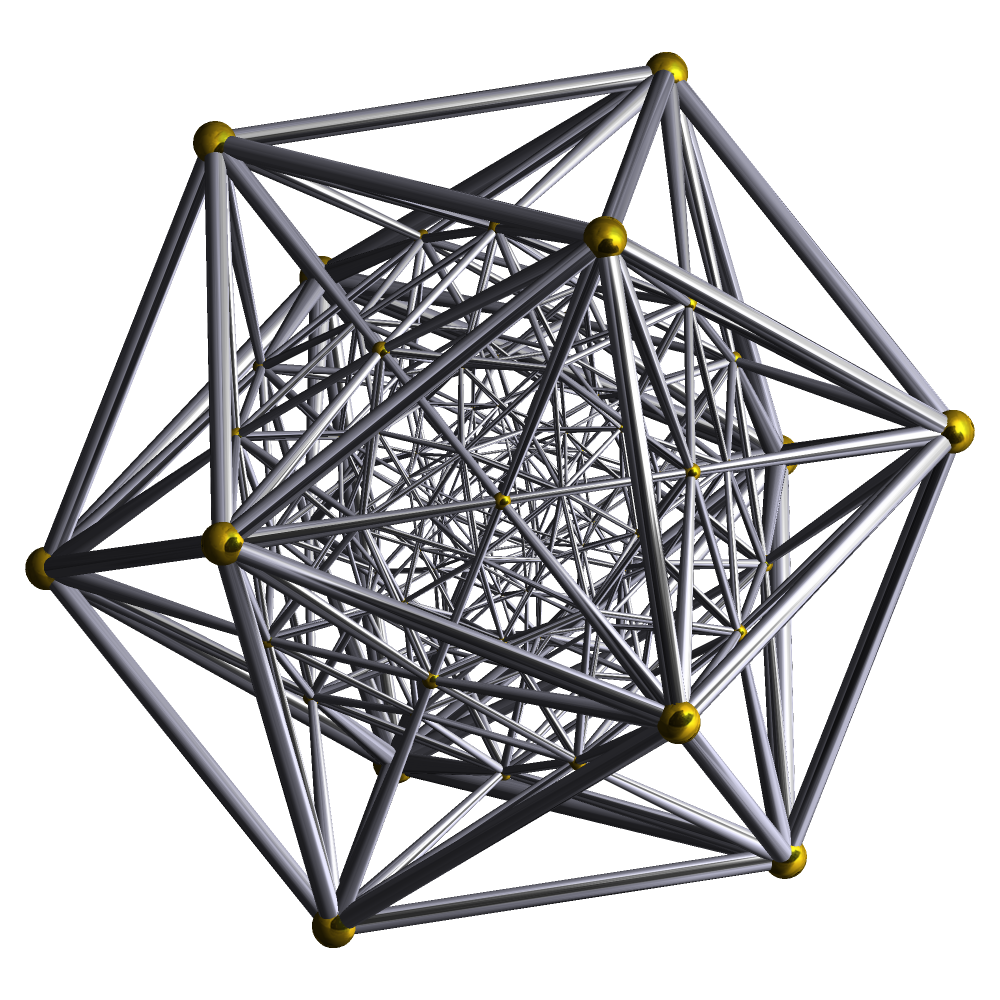Explicitly, if we identify $\mathbb{H}$ with $\mathbb{R}^4$, the elements of $\Gamma$ are the points

$\displaystyle{ (\pm \textstyle{\frac{1}{2}}, \pm \textstyle{\frac{1}{2}},\pm \textstyle{\frac{1}{2}},\pm \textstyle{\frac{1}{2}}) }$

$\displaystyle{ (\pm 1, 0, 0, 0) }$

$\displaystyle{ \textstyle{\frac{1}{2}} (\pm \Phi, \pm 1 , \pm 1/\Phi, 0 ),}$

and those obtained from these by even permutations of the coordinates. Since these points are closed under multiplication, if we take integral linear combinations of them we get a subring of the quaternions:

$\displaystyle{ \mathbb{I} = \{ \sum_{q \in \Gamma} a_q q : \; a_q \in \mathbb{Z} \} \subset \mathbb{H} .}$

Conway and Sloane [CS] call this the ring of icosians. The icosians are not a lattice in the quaternions: they are dense. However, any icosian is of the form $a + bi + cj + dk$ where $a,b,c$, and $d$ live in the golden field

$\displaystyle{ \mathbb{Q}(\sqrt{5}) = \{ x + \sqrt{5} y : \; x,y \in \mathbb{Q}\} }$

Thus we can think of an icosian as an 8-tuple of rational numbers. Such 8-tuples form a lattice in 8 dimensions.

In fact we can put a norm on the icosians as follows. For $q \in \mathbb{I}$ the usual quaternionic norm has

$\displaystyle{ |q|^2 = x + \sqrt{5} y }$

for some rational numbers $x$ and $y$, but we can define a new norm on $\mathbb{I}$ by setting

$\displaystyle{ \|q\|^2 = x + y }$

With respect to this new norm, the icosians form a lattice that fits isometrically in 8-dimensional Euclidean space. And this is none other than $\mathrm{E}_8$!

### Klein’s Icosahedral Function

Not only is the $\mathrm{E}_8$ lattice hiding in the icosahedron; so is the $\mathrm{E}_8$ Dynkin diagram. The space of all regular icosahedra of arbitrary size centered at the origin has a singularity, which corresponds to a degenerate special case: the icosahedron of zero size. If we resolve this singularity in a minimal way we get eight Riemann spheres, intersecting in a pattern described by the $\mathrm{E}_8$ Dynkin diagram!

This remarkable story starts around 1884 with Felix Klein’s Lectures on the Icosahedron [Kl]. In this work he inscribed an icosahedron in the Riemann sphere, $\mathbb{C}\mathrm{P}^1$. He thus got the icosahedron’s symmetry group, $\mathrm{A}_5$, to act as conformal transformations of $\mathbb{C}\mathrm{P}^1$ — indeed, rotations. He then found a rational function of one complex variable that is invariant under all these transformations. This function equals $0$ at the centers of the icosahedron’s faces, 1 at the midpoints of its edges, and $\infty$ at its vertices.

Here is Klein’s icosahedral function as drawn by Abdelaziz Nait Merzouk. The color shows its phase, while the contour lines show its magnitude: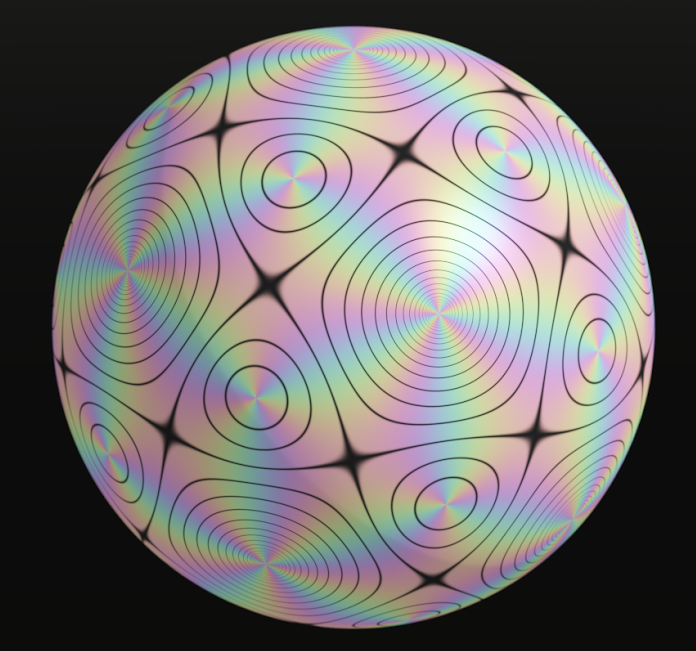We can think of Klein’s icosahedral function as a branched cover of the Riemann sphere by itself with 60 sheets:

$\displaystyle{ \mathcal{I} \colon \mathbb{C}\mathrm{P}^1 \to \mathbb{C}\mathrm{P}^1 .}$

Indeed, $\mathrm{A}_5$ acts on $\mathbb{C}\mathrm{P}^1$, and the quotient space $\mathbb{C}\mathrm{P}^1/\mathrm{A}_5$ is isomorphic to $\mathbb{C}\mathrm{P}^1$ again. The function $\mathcal{I}$ gives an explicit formula for the quotient map $\mathbb{C}\mathrm{P}^1 \to \mathbb{C}\mathrm{P}^1/\mathrm{A}_5 \cong \mathbb{C}\mathrm{P}^1$.

Klein managed to reduce solving the quintic to the problem of solving the equation $\mathcal{I}(z) = w$ for $z$. A modern exposition of this result is Shurman’s Geometry of the Quintic [Sh]. For a more high-powered approach, see the paper by Nash [N]. Unfortunately, neither of these treatments avoids complicated calculations. But our interest in Klein’s icosahedral function here does not come from its connection to the quintic: instead, we want to see its connection to $\mathrm{E}_8$.

For this we should actually construct Klein’s icosahedral function. To do this, recall that the Riemann sphere $\mathbb{C}\mathrm{P}^1$ is the space of 1-dimensional linear subspaces of $\mathbb{C}^2$. Let us work directly with $\mathbb{C}^2$. While $\mathrm{SO}(3)$ acts on $\mathbb{C}\mathrm{P}^1$, this comes from an action of this group’s double cover $\mathrm{SU}(2)$ on $\mathbb{C}^2$. As we have seen, the rotational symmetry group of the icosahedron, $\mathrm{A}_5 \subset \mathrm{SO}(3)$, is double covered by the binary icosahedral group $\Gamma \subset \mathrm{SU}(2)$. To build an $\mathrm{A}_5$-invariant rational function on $\mathbb{C}\mathrm{P}^1$, we should thus look for $\Gamma$-invariant homogeneous polynomials on $\mathbb{C}^2$.

It is easy to construct three such polynomials:

$V$, of degree $12$, vanishing on the 1d subspaces corresponding to icosahedron vertices.

$E$, of degree $30$, vanishing on the 1d subspaces corresponding to icosahedron edge midpoints.

$F$, of degree $20$, vanishing on the 1d subspaces corresponding to icosahedron face centers.

Remember, we have embedded the icosahedron in $\mathbb{C}\mathrm{P}^1$, and each point in $\mathbb{C}\mathrm{P}^1$ is a 1-dimensional subspace of $\mathbb{C}^2$, so each icosahedron vertex determines such a subspace, and there is a linear function on $\mathbb{C}^2$, unique up to a constant factor, that vanishes on this subspace. The icosahedron has $12$ vertices, so we get $12$ linear functions this way. Multiplying them gives $V$, a homogeneous polynomial of degree $12$ on $\mathbb{C}^2$ that vanishes on all the subspaces corresponding to icosahedron vertices! The same trick gives $E$, which has degree $30$ because the icosahedron has $30$ edges, and $F$, which has degree $20$ because the icosahedron has $20$ faces.

A bit of work is required to check that $V,E$ and $F$ are invariant under $\Gamma$, instead of changing by constant factors under group transformations. Indeed, if we had copied this construction using a tetrahedron or octahedron, this would not be the case. For details, see Shurman’s book [Sh], which is free online, or van Hoboken’s nice thesis [VH].

Since both $F^3$ and $V^5$ have degree $60$, $F^3/V^5$ is homogeneous of degree zero, so it defines a rational function $\mathcal{I} \colon \mathbb{C}\mathrm{P}^1 \to \mathbb{C}\mathrm{P}^1$. This function is invariant under $\mathrm{A}_5$ because $F$ and $V$ are invariant under $\Gamma$. Since $F$ vanishes at face centers of the icosahedron while $V$ vanishes at vertices, $\mathcal{I} = F^3/V^5$ equals $0$ at face centers and $\infty$ at vertices. Finally, thanks to its invariance property, $\mathcal{I}$ takes the same value at every edge center, so we can normalize $V$ or $F$ to make this value 1. Thus, $\mathcal{I}$ has precisely the properties required of Klein’s icosahedral function!

### The Appearance of E8

Now comes the really interesting part. Three polynomials on a 2-dimensional space must obey a relation, and $V,E$, and $F$ obey a very pretty one, at least after we normalize them correctly:

$\displaystyle{ V^5 + E^2 + F^3 = 0. }$

We could guess this relation simply by noting that each term must have the same degree. Every $\Gamma$-invariant polynomial on $\mathbb{C}^2$ is a polynomial in $V, E$ and $F$, and indeed

$\displaystyle{ \mathbb{C}^2 / \Gamma \cong \{ (V,E,F) \in \mathbb{C}^3 \colon \; V^5 + E^2 + F^3 = 0 \} . }$

This complex surface is smooth except at $V = E = F = 0$, where it has a singularity. And hiding in this singularity is $\mathrm{E}_8$!

To see this, we need to ‘resolve’ the singularity. Roughly, this means that we find a smooth complex surface $S$ and an onto map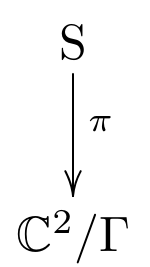that is one-to-one away from the singularity. (More precisely, if $X$ is an algebraic variety with singular points $X_{\mathrm{sing}} \subset X$, $\pi \colon S \to X$ is a resolution of $X$ if $S$ is smooth, $\pi$ is proper, $\pi^{-1}(X - X_{sing})$ is dense in $S$, and $\pi$ is an isomorphism between $\pi^{-1}(X - X_{sing})$ and $X - X_{sing}$. For more details see Lamotke’s book [L].)

There are many such resolutions, but one minimal resolution, meaning that all others factor uniquely through this one: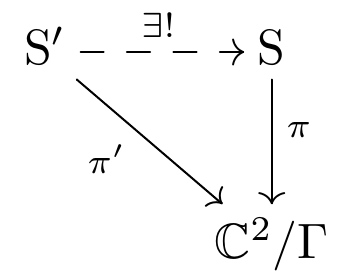What sits above the singularity in this minimal resolution? Eight copies of the Riemann sphere $\mathbb{C}\mathrm{P}^1$, one for each dot here: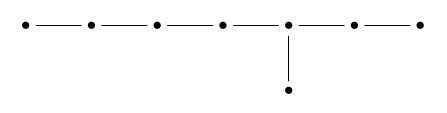Two of these $\mathbb{C}\mathrm{P}^1$s intersect in a point if their dots are connected by an edge: otherwise they are disjoint.

This amazing fact was discovered by Patrick Du Val in 1934 [DV]. Why is it true? Alas, there is not enough room in the margin, or even in the entire blog article, to explain this. The books by Kirillov [Ki] and Lamotke [L] fill in the details. But here is a clue. The $\mathrm{E}_8$ Dynkin diagram has ‘legs’ of lengths $5, 2$ and $3$: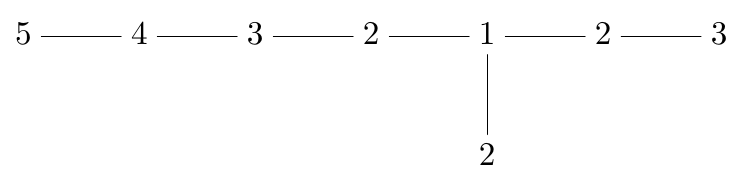On the other hand,

$\displaystyle{ \mathrm{A}_5 \cong \langle v, e, f | v^5 = e^2 = f^3 = v e f = 1 \rangle }$

where in terms of the rotational symmetries of the icosahedron:

$v$ is a $1/5$ turn around some vertex of the icosahedron,

$e$ is a $1/2$ turn around the center of an edge touching that vertex,

$f$ is a $1/3$ turn around the center of a face touching that vertex,

and we must choose the sense of these rotations correctly to obtain $v e f = 1$. To get a presentation of the binary icosahedral group we drop one relation:

$\displaystyle{ \Gamma \cong \langle v, e, f | v^5 = e^2 = f^3 = v e f \rangle }$

The dots in the $\mathrm{E}_8$ Dynkin diagram correspond naturally to conjugacy classes in $\Gamma$, not counting the conjugacy class of the central element $-1$. Each of these conjugacy classes, in turn, gives a copy of $\mathbb{C}\mathrm{P}^1$ in the minimal resolution of $\mathbb{C}^2/\Gamma$.

Not only the $\mathrm{E}_8$ Dynkin diagram, but also the $\mathrm{E}_8$ lattice, can be found in the minimal resolution of $\mathbb{C}^2/\Gamma$. Topologically, this space is a 4-dimensional manifold. Its real second homology group is an 8-dimensional vector space with an inner product given by the intersection pairing. The integral second homology is a lattice in this vector space spanned by the 8 copies of $\mathbb{C}P^1$ we have just seen—and it is a copy of the $\mathrm{E}_8$ lattice [KS].

But let us turn to a more basic question: what is $\mathbb{C}^2/\Gamma$ like as a topological space? To tackle this, first note that we can identify a pair of complex numbers with a single quaternion, and this gives a homeomorphism

$\mathbb{C}^2/\Gamma \cong \mathbb{H}/\Gamma$

where we let $\Gamma$ act by right multiplication on $\mathbb{H}$. So, it suffices to understand $\mathbb{H}/\Gamma$.

Next, note that sitting inside $\mathbb{H}/\Gamma$ are the points coming from the unit sphere in $\mathbb{H}$. These points form the 3-dimensional manifold $\mathrm{SU}(2)/\Gamma$, which is called the Poincaré homology 3-sphere [KS]. This is a wonderful thing in its own right: Poincaré discovered it as a counterexample to his guess that any compact 3-manifold with the same homology as a 3-sphere is actually diffeomorphic to the 3-sphere, and it is deeply connected to $\mathrm{E}_8$. But for our purposes, what matters is that we can think of this manifold in another way, since we have a diffeomorphism

$\mathrm{SU}(2)/\Gamma \cong \mathrm{SO}(3)/\mathrm{A}_5.$

The latter is just the space of all icosahedra inscribed in the unit sphere in 3d space, where we count two as the same if they differ by a rotational symmetry.

This is a nice description of the points of $\mathbb{H}/\Gamma$ coming from points in the unit sphere of $\H$. But every quaternion lies in some sphere centered at the origin of $\mathbb{H}$, of possibly zero radius. It follows that $\mathbb{C}^2/\Gamma \cong \mathbb{H}/\Gamma$ is the space of all icosahedra centered at the origin of 3d space — of arbitrary size, including a degenerate icosahedron of zero size. This degenerate icosahedron is the singular point in $\mathbb{C}^2/\Gamma$. This is where $\E_8$ is hiding.

Clearly much has been left unexplained in this brief account. Most of the missing details can be found in the references. But it remains unknown — at least to me — how the two constructions of $\mathrm{E}_8$ from the icosahedron fit together in a unified picture.

Recall what we did. First we took the binary icosahedral group $\Gamma \subset \mathbb{H}$, took integer linear combinations of its elements, thought of these as forming a lattice in an 8-dimensional rational vector space with a natural norm, and discovered that this lattice is a copy of the $\mathrm{E}_8$ lattice. Then we took $\mathbb{C}^2/\Gamma \cong \mathbb{H}/\Gamma$, took its minimal resolution, and found that the integral 2nd homology of this space, equipped with its natural inner product, is a copy of the $\mathrm{E}_8$ lattice. From the same ingredients we built the same lattice in two very different ways! How are these constructions connected? This puzzle deserves a nice solution.

#### Acknowledgements

I thank Tong Yang for inviting me to speak on this topic at the Annual General Meeting of the Hong Kong Mathematical Society on May 20, 2017, and Guowu Meng for hosting me at the HKUST while I prepared that talk. I also thank the many people, too numerous to accurately list, who have helped me understand these topics over the years.

#### Bibliography

[CS] J. H. Conway and N. J. A. Sloane, Sphere Packings, Lattices and Groups, Springer, Berlin, 2013.

[DV] P. du Val, On isolated singularities of surfaces which do not affect the conditions of adjunction, I, II and III, Proc. Camb. Phil. Soc. 30, 453–459, 460–465, 483–491.

[KS] R. Kirby and M. Scharlemann, Eight faces of the Poincaré homology 3-sphere, Usp. Mat. Nauk. 37 (1982), 139–159. Available at https://tinyurl.com/ybrn4pjq.

[Ki] A. Kirillov, Quiver Representations and Quiver Varieties, AMS, Providence, Rhode Island, 2016.

[Kl] F. Klein, Lectures on the Ikosahedron and the Solution of Equations of the Fifth Degree, Trüubner & Co., London, 1888. Available at https://archive.org/details/cu31924059413439.

[L] K. Lamotke, Regular Solids and Isolated Singularities, Vieweg & Sohn, Braunschweig, 1986.

[N] O. Nash, On Klein’s icosahedral solution of the quintic. Available as arXiv:1308.0955.

[Sh] J. Shurman, Geometry of the Quintic, Wiley, New York, 1997. Available at http://people.reed.edu/~jerry/Quintic/quintic.html.

[Sl] P. Slodowy, Platonic solids, Kleinian singularities, and Lie groups, in Algebraic Geometry, Lecture Notes in Mathematics 1008, Springer, Berlin, 1983, pp. 102–138.

[VH] J. van Hoboken, Platonic Solids, Binary Polyhedral Groups, Kleinian Singularities and Lie Algebras of Type A, D, E, Master’s Thesis, University of Amsterdam, 2002. Available at http://math.ucr.edu/home/baez/joris_van_hoboken_platonic.pdf.

[V] M. Viazovska, The sphere packing problem in dimension 8, Ann. Math. 185 (2017), 991–1015. Available at https://arxiv.org/abs/1603.04246.

Posted at December 11, 2017 1:13 AM UTC

TrackBack URL for this Entry:   https://golem.ph.utexas.edu/cgi-bin/MT-3.0/dxy-tb.fcgi/3000

### Re: The Icosahedron and E8

Now I’ve made more progress… but I’ve realized that the details would never fit in the Newsletter, so I’m afraid anyone interested will have to wait a bit longer.

I’ll be waiting eagerly! Your posts from back in the summer led me to poke around and find a few fun little things, but I never made progress on the central question.

Posted by: Blake Stacey on December 13, 2017 5:14 PM | Permalink | Reply to this

### Re: The Icosahedron and E8

By the way, I’m really sorry that I never responded to your cool notes on $\mathrm{E}_6, \mathrm{E}_7, \mathrm{E}_8$ and positive-operator valued measures! Part of the problem was that I’m not always getting email notifications of comments on my posts here anymore. But mainly I just got distracted, caught up in deadlines.

I’m not sure I would have had anything very intelligent to say, but it can be sort of disheartening to write a bunch of stuff and not get any response.

Well, okay, here’s a question. Near the end of your notes you mention $PSU(3,3)$. This could mean a couple of things: an algebraic group defined over the field $\mathbb{F}_3$ that’s analogous to the 8-dimensional Lie group $PSU(3)$, or the projectivization of the special unitary group of $\mathbb{C}^{3,3}$, that is, a 6-dimensional complex space equipped with a hermitian form of signature $(3,3)$.

I figure it must be the former, since moments later you say it’s almost isomorphic to $\mathrm{G}_2(2)$, which I guess is $\mathrm{G}_2$ defined over $\mathbb{F}_2$. But then you say $\mathrm{G}_2(2)$ is the automorphism group of the ‘octavians’, and that throws me. I don’t know what those ‘octavians’ are… the only thing that fits the data is a version of the octonions defined over $\mathbb{F}_2$. But the algebra of octonions defined over $\mathbb{F}_2$ is a funny thing, since all the signs become irrelevant. I believe it’s just the field $\mathbb{F}_8$.

Posted by: John Baez on December 13, 2017 6:43 PM | Permalink | Reply to this

### Re: The Icosahedron and E8

Ah, sorry. Octavian is the term that Conway and Smith use for the Cayley integers. Quoting On Quaternions and Octonions (2003), chapter 9:

In this chapter, we first find the correct way to define “the integral octonions” and investigate the geometry of the very interesting lattice $E_8$ that they form. […] The elements of O have been called “The Integral Cayley Numbers” since Coxeter’s paper of that title. Later, Coxeter remarked “If I had read F. van der Blij’s ‘History of the octaves’ before writing [that paper], I would have entitled it ‘Integral Octaves.’ ” However, the term “octonion” seems now to have become standard. By way of compromise, we propose to use “octavian” (analogous to “Hurwitzian”) for “octonion integer,” and in further homage to Graves, “Gravesian” for the analogue of “Lipschitzian.”

This is the chapter with Gravesian integers, Kirmse’s Mistake, etc.

Posted by: Blake Stacey on December 13, 2017 7:09 PM | Permalink | Reply to this

### Re: The Icosahedron and E8

Okay, I can sorta see why $G_2$ defined over $\mathbb{F}_2$ is the automorphism group of the octavians, aka Cayley integral octonions. That makes sense now.

Posted by: John Baez on December 13, 2017 10:16 PM | Permalink | Reply to this

### Re: The Icosahedron and E8

I first encountered the group $PSU(3,3)$ via Huangjun Zhu’s PhD thesis, where he defines a group of order 6,048 in terms of two unitary generators, $U_7$ with order 7 and $U_{12}$ with order 12. (See Eq. (10.30) on page 221 for the details.) Constructing the new unitaries $U_a = U_{12} U_7$ and $U_b = U_{12}^2$, one finds that $U_a$ and $U_b$ satisfy the relations for the generators of $PSU(3,3)$ as given in the Atlas of Finite Group Representations, although the Atlas calls this group $U_3(3)$.

The study of this group very rapidly goes off into territory I do not understand, because it shows up in the construction of the Hall–Janko and Rudvalis sporadic simple groups.

Posted by: Blake Stacey on December 13, 2017 7:31 PM | Permalink | Reply to this

### Re: The Icosahedron and E8

Okay—you still haven’t said what the group $PSU(3,3)$ actually is, but everything you say is consistent with my first hypothesis.

Let me say what I think $PSU(3,3)$ is.

For any field $\mathbb{F}$ equipped with an involution $a \mapsto \overline{a}$, we can define the unitary group $\mathrm{U}(n,\mathbb{F})$ to consist of $n \times n$ unitary matrices $U$, meaning those with

$U U^\dagger = 1,$

where

$(U^\dagger)_{i j} = \overline{U}_{j i}$

(this is why we need that involution). We can define the special unitary group $SU(n,\mathbb{F})$ to consist of matrices $U \in \mathrm{U}(n,\mathbb{F})$ with $det(U) = 1$. And we can define the projective special unitary group $PSU(n,\mathbb{F})$ to be $SU(n,\mathbb{F})$ modulo its center, which I believe always consists precisely of the diagonal matrices in $SU(n,\mathbb{F})$.

Finally, we define

$PSU(3,3) = PSU(3,\mathbb{F}_3)$

where we equip $\mathbb{F}_3$ with some involution or other.

I can’t think of a nontrivial involution on $\mathbb{F}_3$ because an involution has to be bijective and it has to preserve 0 and 1, so it has to map $2 \in \mathbb{F}_3$ to itself.

So, I guess we use the trivial involution, which makes me a bit puzzled about why we’re talking about unitary groups in the first place. If we use the trivial involution on our field, a unitary matrix is simply one with $U U^\top = 1$; we usually call these matrices simply orthogonal. (Think of the real case.)

Maybe I’m doing something dumb.

Posted by: John Baez on December 13, 2017 7:49 PM | Permalink | Reply to this

### Re: The Icosahedron and E8

It’s $PSU(3,\mathbb{F}_9)$, or as Conway and Smith put it (p. 134), “the group $U_3(3)$ of “unitary” $3 \times 3$ matrices with entries in the field of order 9.” Why the common notations both say “3” instead of “9,” I don’t know.

Posted by: Blake Stacey on December 13, 2017 9:09 PM | Permalink | Reply to this

### Re: The Icosahedron and E8

Huh. Apparently, the notational ambiguity is confusing enough that Wikipedia remarks on it: Projective unitary group § Finite fields.

Posted by: Blake Stacey on December 13, 2017 9:14 PM | Permalink | Reply to this

### Re: The Icosahedron and E8

Blake wrote:

It’s $PSU(3,\mathbb{F}_9)$, or as Conway and Smith put it (p. 134), “the group $U_3(3)$ of “unitary” $3 \times 3$ matrices with entries in the field of order 9.”

Okay, great!

Why the common notations both say “3” instead of “9,” I don’t know.

Oh, I think I get it. To define unitary matrices we want a field with involution. To get a field with an involution, it’s good to take a field that doesn’t have a square root of $-1$, throw in one (and thus two), and get a bigger field $\mathbb{F}$ whose involution switches these two square roots of $-1$.

If we do this starting with $\mathbb{R}$ we get $\mathbb{F} = \mathbb{C}$ with its usual notion of complex conjugation. If we do this starting with $\mathbb{F}_3$, we get $\mathbb{F} = \mathbb{F}_9$.

In fact my notation is also confusing, because my $SU(n,\mathbb{F})$ is not an algebraic group over $\mathbb{F}$, but only over the smaller field we started with. For example my group $SU(n,\mathbb{C})$ (usually called just $SU(n)$) is not a complex Lie group, just a real one. Similarly my $SU(n,\mathbb{F}_9)$ is only an algebraic group over $\mathbb{F}_3$.

Posted by: John Baez on December 13, 2017 10:07 PM | Permalink | Reply to this

### Re: The Icosahedron and E8

Not specifically a square root of $-1$, just generally a quadratic extension $\mathbb{F}_{q^2}$ of $\mathbb{F}_q$, with the involution being the frobenius automorphism $x\rightarrow x^q$.

Posted by: Tim Silverman on December 20, 2017 10:10 PM | Permalink | Reply to this

### Re: The Icosahedron and E8

Right, I realized that as soon as I hit ‘Post’.

By the way, Tim, I’ve been thinking a lot about the expository articles on math that I’d like to write before I kick the bucket, and one of them might be called “It From Bit”. But unlike the usual meaning of that phrase, I’d want to write about a lot of fun exceptional mathematics that shows up in characteristic 2. I’ll get back to you if I ever get around to this!

(I probably wouldn’t actually use that title, because it’d be too misleading. But as a physicist of sorts, I find the idea of “building complex structures from bits” more exciting than “doing math in characteristic 2”. Just a matter of perspective, really.)

Posted by: John Baez on December 20, 2017 10:43 PM | Permalink | Reply to this

### Re: The Icosahedron and E8

And the plot thickens via del Pezzo surfaces:

A Mysterious Duality

hep-th/0111068

Posted by: Metatron on December 13, 2017 7:10 PM | Permalink | Reply to this

### Re: The Icosahedron and E8

It would be nice if you could give a short summary about the intent of the article and its connection to the topic of the post, for people that don’t speak M-theory/analytic geometry.

Posted by: Alex on December 13, 2017 8:11 PM | Permalink | Reply to this

### Re: The Icosahedron and E8

The article concerns compactifications of M-theory on higher dimensional tori (doughnuts). The observation is that M-theory compactified on a k-dimensional torus $T^k$ corresponds to the complex projective plane $\mathbb{CP}^2$ blown up at k generic points, where k=1,..,8.

Each time a point is blown up to a $\mathbb{CP}^1$ (sphere) giving del Pezzo surface $\mathbb{B}_k$, M-theory is compactified on another circle. This is the so-called Mysterious Duality. The case k=8 corresponds to $T^8$ (8D torus), M-theory in 11D reduced to 3D, so this gives 8 blown up points that become spheres. The group of global diffeomorphisms of the del Pezzo surface is isomorphic to the Weyl group of $E_8$, in this case. (Note: $E_6$ and $E_7$ are recovered for k=6 and k=7)

Compare this k=8 del Pezzo surface $\mathbb{B}_8$ to John’s minimal resolution, with 8 Riemann spheres ($\mathbb{CP}^1$) for each dot in the Dynkin diagram.

Posted by: Metatron on December 14, 2017 6:21 AM | Permalink | Reply to this

### Re: The Icosahedron and E8

Posted by: Alex on December 14, 2017 4:31 PM | Permalink | Reply to this

### Re: The Icosahedron and E8

Metatron, what meaning have you chosen for the phrase “global diffeomorphism” [of the del Pezzo surface]? The group of $C^\infty$ self-maps is infinite-dimensional. The group of complex-analytic automorphisms is finite but always much smaller than the corresponding Weyl group. For $k=8$ it is generically of order $2$.

Posted by: Nick on December 15, 2017 3:45 PM | Permalink | Reply to this

Post a New Comment